# Divide money

Divide 1200 USD at a ratio of 1:2:3:4:5:6:9:10

a =  30
b =  60
c =  90
d =  120
e =  150
f =  180
g =  270
h =  300

### Step-by-step explanation: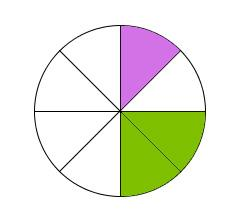Did you find an error or inaccuracy? Feel free to write us. Thank you!Tips to related online calculators
Need help to calculate sum, simplify or multiply fractions? Try our fraction calculator.
Check out our ratio calculator.

## Related math problems and questions:

• Composite ratioJakub, Aneta, and Lenka divided 1342 USD in the ratio 5/2: 3/10: 1/4. How much did Lenka take?
• Kilo candyOne kilogram of chocolate candy cost 10 euros. Kate buys 250 grams and had the 8 pack of candy. How much does cost one bonbon?
• RatiosReduce the numbers: 50 in a 1:2 ratio 111 at a ratio of 2:3 70 at 10:50 560 at a ratio of 3:8
• Babysitting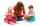The amount that Susan charges per hour for babysitting are directly proportional to the number of children she is watching. She charges \$4.50 for 3 kids. How much would she charge to babysit 5 kids?
• Ratio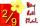Write the ratio with other numbers so that the value is the same: 2: 9
• Apples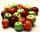Mr. Vesely give to the market his own 48 kg of apples, 40 kg from Mr. Kovar and 72 kg of Mr. Novak. Grossed a total of 1,920 CZK. How must divide income money for apples?
• DivideDivide area of rectangles with dimensions 32m and 10m by the ratio 7: 9. What area corresponds to a smaller section?
• PamelaLara Pamela spends her salary of 3000 for food, clothing, recreation and savings, which are in the ratio of 48:20:15:37, respectively. How much does he spend for savings?
• Change the numbers in the ratio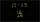Change the numbers 29, 38, and 43 in a 3: 4 ratio.
• BonusThe gross wage was 1323 USD including 25% bonus. How many USD were bonuses?
• Honza + Alice + Tonda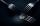Honza + Alice + Tonda have a total of 111 USD. The ratio between Honza and Alena is 5:6 and the ratio between Alice and Tonda is 4:5 How many money have each of them?
• Money split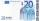Anton and Ferko got 2,500 euros together. The amount was split in a 1:4 ratio. How much did each get?
• Moneys in triple ratioMilan, John and Lili have a total 344 euros. Their amounts are in the ratio 1:2:5. Determine how much each of them has?
• String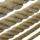Divide the string length 800 cm in ratio 1: 5
• Wage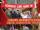Increasing the minimum wage while maintaining the other parameters of the system, unemployment will be:
• Dividing moneyJane and Silvia are to divide 1200 euros in a ratio of 19:11. How many euros does Jane have?
• Ratio6 numbers are in the ratio 1:5:1:5:5:5. Their sum is 242. What are the numbers?